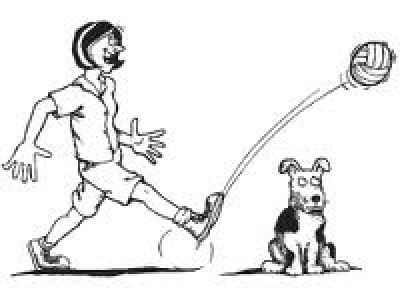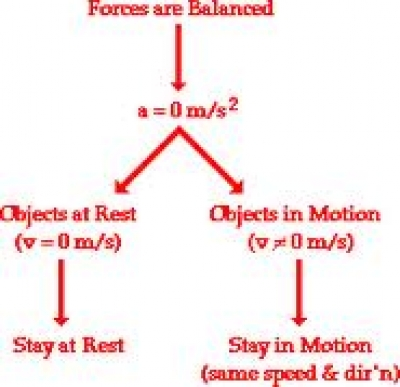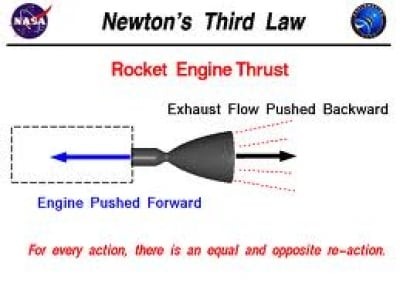# Newtons Laws of Motion Simplified As a Part of Classical Mechanics

Knoji reviews products and up-and-coming brands we think you'll love. In certain cases, we may receive a commission from brands mentioned in our guides. Learn more.
Newtons Laws of motion have universal applicability and understanding them is of paramount importance in today's world

Sir Isaac Newton is considered universally as one of the greatest physicists of all time in the history of science. His fame rests on his laws of motion that brought about a quantum change in theories of classical mechanics. Before we explain the laws of motion (they are 3 in number) it is important to understand three concepts that form part of these laws of motion.

a) Velocity. We all understand the word speed. It is the rate at which a body say a car travels and is usually measured in miles per hour or kilometers per hour. But in classical mechanics the word speed is replaced with velocity and is given a direction as well. Thus representation of speed with direction is velocity. It is measured in feet per second or meters per second in a specified direction. Remember velocity must have direction and is represented by an arrow, showing the direction. It is represented by the letter v which is termed the initial velocity and u which represents the final velocity.

b) Acceleration. A body starts with an initial velocity which may be zero and then speeds to final velocity in a particular direction. This rate of change in velocity of a body from 0 to say 60 ft per second is referred to as acceleration. In case a body continues at a particular velocity, it is referred to as constant acceleration. Acceleration is represented by the letter a.It must be understood that any change in acceleration is dependent on a force that acts on the body.In a simple example a car which starts from rest and reaches a particular velocity is because of the external force, which in this case is supplied by the engine.

c) Force. For a body to move from a stated position say rest, some external power must act on it. This is referred to as force and is pressure exerted on a body that compels it to change its state of rest or motion. Force is directly related to mass, which in simple terms is the weight of a body.

Having understood the basic concept of the three fundamental parameters of classical mechanics we can understand Newton’s Laws of Motion. The 3 Laws of motion in simple language are as follows.

a) The first law states that a body continues in its state of rest or motion unless acted upon by an external force. Thus a football will remain static at a point on the playing field unless acted upon by an external force which in this case is a kick by the player. The ball while in motion is again acted upon by external forces like air and gravity. Air retards the movement of the ball and ultimately brings it back to rest, while the force of gravity pulls the the ball down, till it again comes to rest.By logic in case there was a vacuum and no air the ball would continue its motion . Newton thus introduced a revolutionary concept. This law is also called the Law of Inertia. The mathematical representation of this law is by the famous equation

S=ut+1/2at(square)

s- distance covered

u- initial velocity

t- time elapsed

a- Acceleration

.are other derivatives of this equation, but for a understanding of the first law this equation is a good illustration.

b- The Second Law of motion is the heart of these laws and has given an equation that is as famous There as Einstein’s E=MC(square) This equation is F=M x A, where F is the force and M the mass and A the acceleration. This equation id derived from the second law which states that rate of change of momentum of a body is proportional to the applied force and takes place in the direction of the force.Thus Newton put forward a revolutionary concept that stated that a force exerted is directly proportional to the mass of a body and its acceleration. Thus a large stone falling will generate a greater force than say a tennis ball as the masses of the bodies differ. The diagram above illustrates the effect of force on a body.

c- The third law of motion states that to each and every action there is a reaction. Thus when a rifle is fired , the bullet moves forward, but the gun exerts a force on the shooter in the form of the recoil. The law is also applicable to all actions in the universe. It has particular applicability in the field of rocketry. A rocket moving up exerts a force on the earth, which in turn also exerts a force on the rocket. The rocket can escape from this force only after overcoming the force exerted to it and is the escape velocity. Once that is achieved the rocket moves into space.The Laws of motion as enunciated by Newton have a direct bearing on everyday life. He put forward these laws in his book Principia in 1687. Newton’s laws had a limitation as they are applicable in a particular frame and though fundamentally correct needed to be fine tuned by Bohr and Einstein